# Probabilistic programming with Pyro and conclusive proof that Poland was robbed at the 2018 Chess Olympiad

Yes. I know what you’re thinking. “Chess is the most important sport on the planet. Perhaps the most important activity there is. If you claim that Poland played better than what their fourth place indicates, you’d better have proof. You’d. Better. Get. This. Right.”

This will be a long post, wherein I

1. Explain “Why Pyro”
2. Describe chess and the Olympiad in excruciating detail
3. Finally get to the code part, where we model the result of a chess tournament
4. Solve the model in various ways
6. Draw conclusions!

## Pyro

To get this right, I’d like to use probabilistic programming and Pyro.

At Tenfifty, we like Pytorch. Compared to Tensorflow, the eager execution feels much more like Python programming. When I have done probabilistic programming in the past, I have generally used PyMC3, which is nice enough. However, being built on top of Theano, it does suffer from the same problem as Tensorflow. The compiling and lazy execution can lead to strange error messages and a suboptimal REPL experience. Also Theano is not developed anymore.

Enter Pyro. Pyro is a new probabilistic programming library, built on top of Pytorch. It is still in alpha, but seems to work well. Pytorch was recently released in a 1.0 preview, which led me to do this experiment in Pytorch 1.0 (preview) and the branch of Pyro that supports Pytorch 1.0.

I learned Pyro as I worked on this, so I may have gotten some details wrong. Probably not, though.

## Chess background

So, what will we use Pyro for? Recently, the 2018 Chess Olympiad was concluded. It was generally exciting, as chess tends to be, but lead to the type of discussions that seems to be the point of sports – “Did the right team win?”, “Is the system fair?”, “Could this perhaps be ruined with enough math and programming?”.

You see, the Chess Olympiad is played as a team sport. Four players from one country play four players from another country. Four games in total and you sum up the results (draw counts as 0.5). 184 teams entered the Open Section (there is also a Women’s Section, but since everyone is eligible to play in the Open, I will focus on that one), almost all of them from separate countries. They play 11 rounds. Each round, countries get paired in matches against other countries with the same score so far. This system is used in many sports and is called a Swiss pairing. The devil is in the details, however, and this year the pairing was sort of odd.

After the tournament had concluded, there were three teams with the same number of wins. Who was the tournament winner? This is decided via a complex calculation of tiebreaks, using the results of the teams that each of the three teams have played, to see who had the toughest opposition. My first question, then, is Was this procedure fair?

The second question regards Poland. Poland was the tournament surprise. For nine rounds they played the best chess and were the tournament leaders. They met all the best teams and didn’t lose a single match, until round 10. After the full eleven rounds, they placed fourth. No medal for Poland. Strong grandmasters and people in general on Twitter seemed to regard this as a scandal. Had Poland not shown that they were worthy of a medal? They had played the strongest opposition by far and faltered just at the finish line. The system was unfair! Should Poland have gotten a medal?

Being a curmudgeonly and generally unpleasant sort of fellow, I though to myself “Bah. Humbug. They just favor the underdog with their filthy emotions. I bet fourth place was even too good for that uppity Polish team. Let’s spend several hours proving that someone on the Internet is wrong.” Also I thought it would serve humanity to show that Sweden is better than Norway at chess.

## The Elo system

60 years ago, Arpad Elo devised the Elo system. The basic idea is that a player’s strength can be expressed through a number. In a particular game, the player’s (unmeasurable) performance is drawn from a normal distribution around the player’s rating with a standard deviance such that 100 points difference in rating should lead to a 64% score over time.

Below, I have chosen to write the formula directly and return the probabilities for player 1-win, draw and player 2-win. We don’t have to do this, though. Instead, I could have encoded how I believe the result is generated from probability distributions, as described above. Elo didn’t specify what percentage of the expected score will come from draws and what part will come from decided games, so I have left this as a parameter.

I think the point of a competition is to estimate the true Elo of the participants, at this particular point in time, using no knowledge of their prior performance. This means that a good pairing system should be aimed at resolving this question and that a good tiebreak system should favour the player with the best estimated performance. From a sporting point of view it is also good if a tiebreak system is easy to understand, so I don’t actually propose this computation as a better method in practice. Just as a measure.

In :
import torch

def chess_game(p1_elo, p2_elo, draw_ratio):
diff = p1_elo - p2_elo
p1_winp = 1 / (1 + 10 ** (-diff/400))
p2_winp = 1 - p1_winp
minp = 1 / (1 + 10 ** (abs(diff)/400))
drawp = minp * torch.clamp(draw_ratio, 0.01, 0.99)



This is just a normal function that takes arguments and produces a 2D array of three probabilites (win1, draw, win2) per row in p1_elo and p2_elo. We write our functions for tensors rather than floats, so that we can calculate them for all games in a tournament (see below) at the same time.

Below we test it for two games 1800 vs 2000 and 1800 vs 1600. The total score, $win \times \frac{draw}{2}$, for the first player should be the same as the total score for the second in the second example, even though we tried different settings for draw_ratio.

In :
chess_game(torch.tensor([1800.0, 1800]),
torch.tensor([2000.0, 1600]),
torch.tensor([0.5, 0.33]))

Out:
tensor([[0.1201, 0.2403, 0.6396],
[0.6805, 0.1586, 0.1610]])

OK, looks good. In the second example we get less draws, since we gave a lower draw_ratio, and the higher rated player always have a higher win ratio.

## The Tournament

Now, lets define a Tournament. A Tournament takes two tensors with players, p1s and p2s, each containing integers that identify each player. Finally it takes a tensor with results in those games. 0 for player_1-win, 1 for draw and 2 for player_2-win.

We also define a model. The model defines priors for the two unknown parameters which we are trying to find.

1. The true draw_ratio
2. A tensor of the true Elo rating for each player.

The pyro.sample function is used to tell Pyro that this is an unknown parameter. Since I don’t know anything about the tournament, I give the elos a wide prior, centered around 0 for each player, with a standard deviation of 300. This basically says that I don’t know what the rating is, but I’d be very surprised if the difference between players is more than 1000, and it is not going to be 10000.

We then send in the elos and the draw_ratio to our chess_game function and get a bunch of probabilities. Finally, we define a result and say that this parameter is observed. Pyro can use the observed data (the results of the game) to infer the most likely parameter settings.

Ignore the two guide methods for now. Also ignore why it says “.independent(1)” and “with pyro.iarange..” for a little while.

In :
import pyro
import pyro.distributions as dist

pyro.enable_validation(True)

class Tournament:
def __init__(self, p1s, p2s, results):
self.p1s = torch.tensor(p1s)
self.p2s = torch.tensor(p2s)
self.results = torch.tensor(results)
self.n_players = max(self.p1s.max(), self.p2s.max()).item() + 1

def model(self):
draw_ratio = pyro.sample('draw_ratio', dist.Beta(5, 5))
elodist = dist.Normal(torch.zeros(self.n_players), 300)
elos = pyro.sample('elos', elodist.independent(1))
p = chess_game(elos[self.p1s], elos[self.p2s], draw_ratio)

with pyro.iarange("data_iarange", len(self.results)):
result = pyro.sample('result',
dist.Categorical(p),
obs=self.results)

def MAP_guide(self):
pd = pyro.param('pdraw_ratio', torch.rand(1) * 0.6 + 0.3)
pyro.sample('draw_ratio', dist.Delta(pd))
elo_guess = torch.rand(self.n_players) * 200 - 100
elos_mu = pyro.param("elos_mu", elo_guess)
pyro.sample('elos', dist.Delta(elos_mu).independent(1))

def norm_guide(self):
a = pyro.param('draw_alpha', torch.rand(1) * 10)
b = pyro.param('draw_beta', torch.rand(1) * 10)
pyro.sample('draw_ratio', dist.Beta(a, b))
elo_guess = torch.rand(self.n_players) * 200 - 100
sd_guess = torch.rand(self.n_players) * 200
elos_mu = pyro.param("elos_mu", elo_guess)
elos_sd = torch.clamp(pyro.param("elos_sd", sd_guess, 1.0))
elo_dist = dist.Normal(elos_mu, elos_sd)
pyro.sample('elos', elo_dist.independent(1))


Now, the magic begins. We gave a starting prior on our parameters, just giving the shape and size of our ignorance. Pyro can now use the results of the games to infer the posterior of our parameters. The posterior is statistics talk for what we should believe after the data, just as prior is what we believe before the data. The more data we have, the more the posterior will differ from the prior.

There are three main ways of calculating the posterior. Presented in order of increasing CPU usage.

### 1. Maximum a posteriori or “MAP”

MAP means that we are just interested in the most probable combination of parameters. The peak of our multi-dimensional distribution. A MAP is the cheapest and simplest to calculate, but the less data you have, the more interested you should be in how uncertain you are about the parameters. The only thing you need to calculate this is a decent starting point. This is what we define in MAP_guide, above.

Unlike PyMC3, Pyro has no simple way of calculating a MAP. We have to pretend that it is a SVI-calculation (see below), where each parameter is Delta distributed (just a point). The torch.rand-things are just starting points for the optimization.

### 2. Stochastic variational inference

In SVI, you are interested in how certain you are of your posterior. This is a somewhat harder problem. To help solve this, we give the algorithm two more things (this is what a norm_guide does, above), the approximate shape of our posterior and which parameters can be assumed to be independent of each other. The independence information can help the algorithm to solve the problem more efficiently.

The “with …” that I asked you to ignore earlier, just says that all my results can be assumed to be independent. A common assumption. The “independent(1)” says that the elo tensor is also independent along the first dimension. We don’t have to give this information, but it helps SVI. In theory – I didn’t check.

### 3. Markov Chain Monte Carlo

In MCMC, we don’t make any assumptions about the posterior distribution. We just ask the algorithm to find a good starting point (e.g MAP), jump around and sample points according to a criterion that gives us a good estimate of our posterior shape. In SVI, we often assume that our posterior parameter belief will be bell shaped around some midpoint, but MCMC can tell us, for example, if our posterior belief should be multi-modal (several peaks), or if the distribution is skewed to one side around our peak.

MCMC is really slow, though. And it suffers from the problem that the samples can be correlated. And the more dimensions you add (players in your tournament), the slower it gets. Luckily, we have something called Hamiltonian Monte Carlo – HMC, which instead of jumping around randomly in our probability space, slides around with momentum, like a frictionless puck on an ice landscape with hills and valleys. This method turns out to be less likely to get stuck in a valley and much more efficient in many dimensions, if you have continuous parameters (if you have discrete parameters, you are out of luck – use MCMC or reparameterize your problem).

The problem with HMC is that it is finicky with parameters, but we are in luck again! NUTS-sampling was invented, which takes care of setting the parameters automatically.

Simplifying somewhat, we can say that machine learning is normally interested in just MAP – the point estimate. For example standard deep learning. Sometimes someone wants to make a neural network where the weights have uncertainty, which can for example help in transfer learning. HMC is usually (but not always!) too slow for this, so they say that the posterior weights are normally distributed, use SVI and get on with their lives.

The best approximation of the true posterior is given by HMC, so if time permits, that is what we will use.

## Our first tournament

To test the inference algorithms, let’s start with a simple tournament with three players. First p0 plays p1 (draw!), then p0 plays p2 (win for p0!) and finally p1 draws p2. p0 got 1.5 points, p1 got 1.0 points and p2 got 0.5.

A typical sign that we need statistical learning and not machine learning is that we are optimizing four parameters (three elos and a draw ratio) using only three data points. That is why we need some priors (the less data we have, the more important are the priors) and we need to check our uncertainty in the end, since we will still be very uncertain after three data points.

In :
easy_tourney = Tournament([0, 0, 1], [1, 2, 2], [1, 0, 1])


If we want, we can use one of our guides to define a simple loss function, whose parameters can be optimized in the usual way. The parameters are stored and optimized in a global object (ugly, IMHO, why not in a with-context or something?), so we need to clear the parameter store between runs.

In :
ps = pyro.get_param_store()
ps.clear()
loss = pyro.infer.Trace_ELBO()
[loss.loss(easy_tourney.model, easy_tourney.norm_guide) for _ in range(20)]

Out:
[5.159919738769531,
5.960174560546875,
7.503453254699707,
6.657198905944824,
6.24017333984375,
7.232036590576172,
7.073713302612305,
8.353660583496094,
8.430072784423828,
6.119638442993164,
6.748605728149414,
8.007732391357422,
6.507745742797852,
7.801944732666016,
7.93726921081543,
7.528817176818848,
7.462062835693359,
7.116822242736816,
7.683196067810059,
4.05120849609375]

Above, we have 20 runs of our loss function with the same parameters. It is non-deterministic, because in our guide where it says pyro.sample, a sample is drawn from the distributions whose parameters we specify. This means that the loss function is really noisy (more observations would make it less noisy, I think), which is a challenge for any optimization method. MAP and SVI optimization is done using the same methods that we use for neural networks. In my experiments, Adam repeatedly proved best able to cope with all this noise.

Let’s use Adam to define a function that runs a few epochs, plots the improving loss and prints the parameters in the final solution.

In :
import matplotlib.pyplot as plt
import pyro.optim

def optimize_parameters(model, guide, iterations, print_solution=True):
ps = pyro.get_param_store()
ps.clear()

svi = pyro.infer.SVI(model=model,
guide=guide,
loss=pyro.infer.Trace_ELBO())

losses = []
for t in range(iterations):
newloss = svi.step()
losses.append(svi.step())

if print_solution:
ps = pyro.get_param_store()
print(ps.get_state()['params'])

plt.plot(losses)
plt.xlabel("Epochs")
plt.ylabel("loss")
plt.show()


First we calculate the MAP. When we have just Delta functions in our guide, which will always sample to the same value, there is no noise, and it is easy to optimize.

In :
optimize_parameters(easy_tourney.model, easy_tourney.MAP_guide, 3000)

{'pdraw_ratio': tensor([0.5835], requires_grad=True), 'elos_mu': tensor([ 71.3120,   7.8835, -53.8668], requires_grad=True)}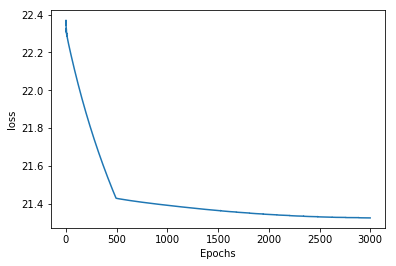OK, $draw\_ratio=0.58$ and $p0-p1=63, p0-p1=135$ (only the differences matter).

In :
optimize_parameters(easy_tourney.model, easy_tourney.norm_guide, 3000)

{'draw_alpha': tensor([6.8134], requires_grad=True), 'draw_beta': tensor([5.9586], requires_grad=True), 'elos_mu': tensor([132.6411,  53.7772, -36.8663], requires_grad=True), 'elos_sd': tensor([169.3705, 144.9115, 171.9290], requires_grad=True)}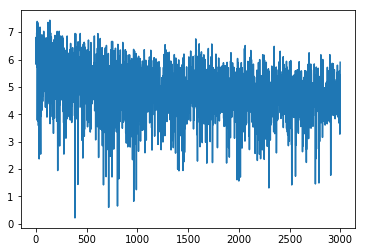Also optimizing for uncertainty turns out much noisier, but we get approximately the same Elo differences, though somewhat larger, and of course a huge uncertainty (the elos_sd tensor) after only three games. The draw_ratio ($\frac{\alpha}{\alpha+\beta}=0.54$) is also basically the same.

Now let’s use HMC to take a few samples and compare. warmup_steps denotes the number of steps that should be discarded because the algorithm has not yet converged yet. We take 300 samples. That is not a huge amount, but OK for now. If we wanted to do things right, we would produce several independent chains of these samples – several runs – to check that they agree.

In :
import pyro.infer.mcmc

nuts_kernel = pyro.infer.mcmc.NUTS(easy_tourney.model,
mcmc_run = pyro.infer.mcmc.MCMC(nuts_kernel,
num_samples=300,
warmup_steps=100).run()


Now we can create an empirical distribution from our trace, that we can sample from and calculate mean as well as plot the posterior.

In :
marginal = pyro.infer.EmpiricalMarginal(mcmc_run, sites="elos")
marginal.mean

Out:
tensor([118.0375,  25.5137, -74.4103])

Now, we can plot the relative elos.

In :
import matplotlib.pyplot as plt

def diff(i1, i2, telo):
return (telo[i1] - telo[i2]).item()

trace = [[diff(0, 1, marginal()) for _ in range(1000)],
[diff(0, 2, marginal()) for _ in range(1000)]]
plt.figure(figsize=(20, 10))
plt.hist(trace, bins=30, label=['p0 - p1', 'p0 - p2'])
plt.title("P(Elo | result)")
plt.xlabel("Relative Elo")
plt.ylabel("#")
plt.legend()
plt.show()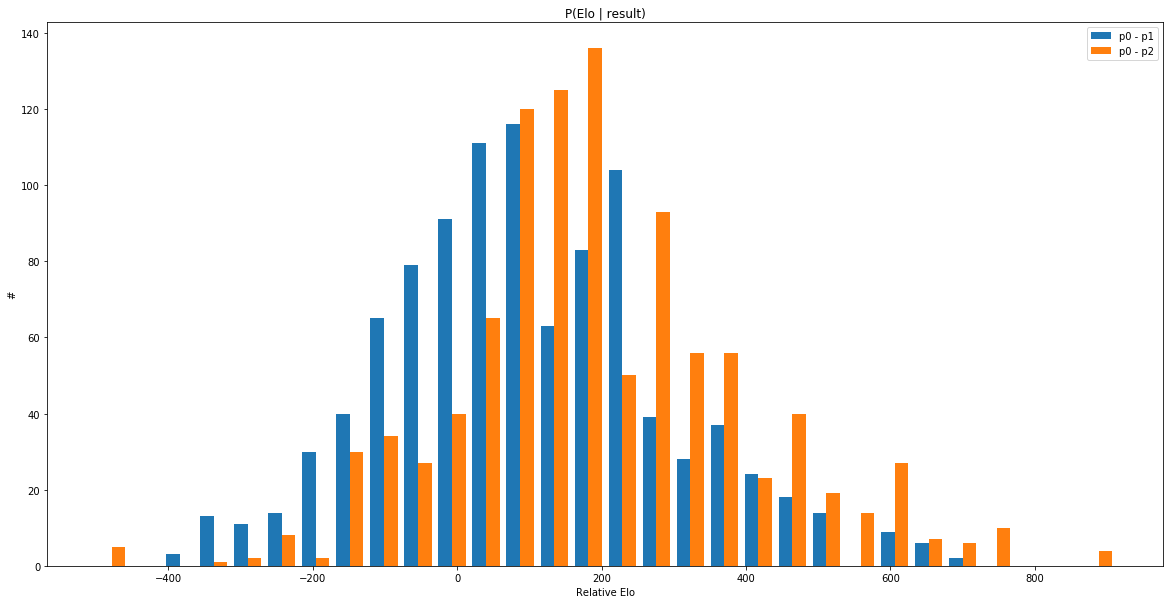From only three games, we can’t really tell who is the best player. We can see that both the distributions go over to the negative side and that they are overlapping, meaning that we don’t know if p1 is better than p2, either.

We can also verify that the draw_ratio is approximately the same, below.

In :
marginal = pyro.infer.EmpiricalMarginal(mcmc_run, sites="draw_ratio")
marginal.mean

Out:
tensor(0.5620)

## A second tournament and a first scandalous result!

Before the main event, analyzing the Chess Olympiad, let’s verify our method one more time.

A common tiebreak in chess tournament is that if all players have played each other and two players are on the same score, you value results against the better players more. The question is if this really implies that you have played better, or if it is more based on a gut feeling of status.

Our tricky tournament has four players. p0 and p1 both got 2 points of 3. p2 got 1.5 and p3 only 0.5. The difference between p0 and p1 is that p0 beat p2 – the “better” player and drew p3, while p1 beat p3 and drew p2. With conventional tiebreaks, p0 would win. Let’s check if this is reasonable.

First with SVI.

In :
p1s = [0, 0, 0, 1, 1, 2]
p2s = [1, 2, 3, 2, 3, 3]
res = [1, 0, 1, 1, 0, 0]
tricky_tourney = Tournament(p1s, p2s, res)

optimize_parameters(tricky_tourney.model, tricky_tourney.norm_guide, 10000)

{'draw_alpha': tensor([8.5943], requires_grad=True), 'draw_beta': tensor([6.3786], requires_grad=True), 'elos_mu': tensor([  63.4225,   81.4776,   11.5082, -176.3225], requires_grad=True), 'elos_sd': tensor([150.9545, 136.3249, 158.8695, 164.9388], requires_grad=True)}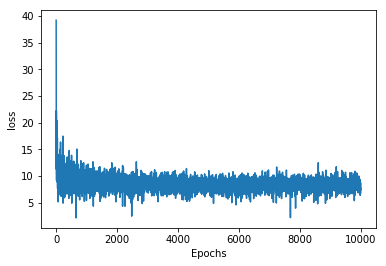Very interesting. Our SVI run says that the expected elo of p1 is higher than for p0. This surprised me and at first I thought that maybe it was just noise, but the result holds when I run SVI several times.

To make sure that this bombshell is confirmed, let’s see if the results hold with a HMC run. This time we use longer warmup and more samples, to make sure that we have the necessary resolution.

In :
nuts_kernel = pyro.infer.mcmc.NUTS(tricky_tourney.model,
mcmc_run_tricky = pyro.infer.mcmc.MCMC(nuts_kernel,
num_samples=1000,
warmup_steps=300).run()

marginal = pyro.infer.EmpiricalMarginal(mcmc_run_tricky, sites="elos")
marginal.mean


Out:
tensor([  63.4488,   87.8144,   14.0909, -174.5992])

Identical results! So it is true, then! The tiebreaks rule are wrong! Seemingly.. In this case.. If Arpad Elo’s models hold true..

If you and another person played the exact same opposition (which is common in closed competitions) and got the same total score, beating a player with less points suggests that you are a stronger player. I think the reason is that my Elo priors, although very wide, give a slight preference for moving the Elo posteriors closer together, compared to a purely frequentist method (which would give no result at all for a player taking 0 points).

We must inform the world.

## The true winner of the Chess Olympiad 2018

In :
import requests
BASE = "http://chess-results.com/tnr368908.aspx?lan=1&art=2&rd=%d&flag=30"

def get_round(i):
r = requests.get(BASE % i)
return r.text

In :
ALLTEAMS = []

In :
import re
RES_REX = re.compile(r'<td class="CR">([^<]+)</td><td class="CRc">[^<]+</td><td class="CRc">[^<]+</td><td class="CRcErg">([^<]+)</td><td class="CRc">:</td><td class="CRcErg">([^<]+)</td><td class="CRc">[^<]+</td><td class="CRc">[^<]+</td><td class="CR">([^<]+)</td>')

def get_results(s):
for x in RES_REX.findall(s):
if x == '&':
res = [0, 1, 3]
else:
win = int(x)
draw = 0
if x[-1] == ';':
draw = 1
res = [win, draw, 4 - win - draw]
if x not in ALLTEAMS:
ALLTEAMS.append(x)
if x[-1] not in ALLTEAMS:
ALLTEAMS.append(x[-1])
yield ALLTEAMS.index(x), ALLTEAMS.index(x[-1]), res

In :
results = []
for i in range(1, 12):
results.extend(list(get_results(get_round(i))))

In :
len(results), len(ALLTEAMS), results

Out:
(1009, 184, (0, 1, [4, 0, 0]))

So, we have 1009 matches of four games each and 184 different teams.

While we’re at it, let’s also get the final standings to compare our results. This link only gives the top 150, but that will be enough for our purposes.

In :
STAND_REX = re.compile(r'</div></td><td class="CR">([^<]+)</td>')
STAND_URL = "http://chess-results.com/tnr368908.aspx?lan=1&art=0&rd=11&flag=30"
r = requests.get(STAND_URL)

print('Final standings:')
standings = STAND_REX.findall(r.text)
standings[:10], len(standings)

Final standings:

Out:
(['China',
'United States of America',
'Russia',
'Poland',
'England',
'India',
'Vietnam',
'Armenia',
'France',
'Ukraine'],
150)
In :
def get_rank(team):
try:
return standings.index(team)
except ValueError:
return -1
TEAMRANKS = [get_rank(team) for team in ALLTEAMS]


Now we convert the data into a format that our Tournament class can accept.

In :
osp1 = []
osp2 = []
osres = []
for p1, p2, res in results:
for i in range(3):
for j in range(res[i]):
osp1.append(p1)
osp2.append(p2)
osres.append(i)
len(osres)

Out:
4036

4036 games. We model each match as four games between two “players” with one Elo each. So the full team gets one combined Elo.

In :
os_tourney = Tournament(osp1, osp2, osres)
optimize_parameters(os_tourney.model,
os_tourney.norm_guide,
20000,
print_solution=False)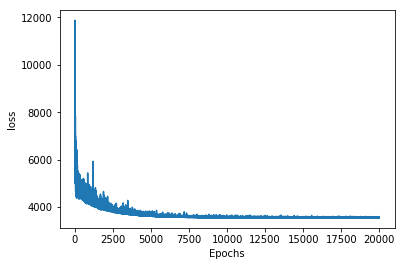And here is a table of our calculated true Elo rating, together with standard deviation, what the “fair” final ranking of the team should be, and what their actual ranking was.

In :
import pandas as pd
ps = pyro.get_param_store()

elos = [round(sol.item()) for sol in ps.get_param('elos_mu')]
sds = [round(solsd.item()) for solsd in ps.get_param('elos_sd')]
svi_ranks = sorted(zip(elos, sds, ALLTEAMS, TEAMRANKS), reverse=True)

pd.DataFrame(svi_ranks[:25],
columns=['Elo', 'Stdev', 'Country', 'Final result'])

Out:
Elo Stdev Country Final result
0 462 50 United States of America 1
1 458 49 Azerbaijan 14
2 457 55 Poland 3
3 444 53 China 0
4 420 53 France 8
5 419 48 Armenia 7
6 418 51 India 5
7 413 52 Russia 2
8 401 49 Ukraine 9
9 395 56 Vietnam 6
10 391 53 Czech Republic 11
11 376 48 England 4
12 367 53 Israel 38
13 366 56 Germany 12
14 364 59 Uzbekistan 15
15 364 53 Croatia 25
16 362 55 Netherlands 39
17 351 50 Iran 16
18 351 49 Spain 24
19 343 57 Georgia 1 27
20 339 54 Romania 26
21 339 52 Hungary 17
22 338 55 Sweden 10
23 322 53 Norway 28
24 312 50 Moldova 30

We can see that our true, fair and generally infallible method is quite close to the actual (zero indexed) final results. We can see that Azerbaijan played much better than their actual final ranking and that Sweden’s position at 10 was way too high, but they still performed clearly – clearly – better than Norway.

On a slightly serious note, we can see by the standard deviation of about 50 and the difference between first and second place of about 4, that we don’t have nearly enough games to actually know which team is the best. There is just way too much randomness in this. The type of random noise that spectators love to interpret as significant.

We don’t quite have the final results yet. I trust HMC slightly more than SVI. The earlier promising results showed that the outcome will probably be the same for SVI and HMC, but let’s run a sampling for a few hours and check. If I used a GPU, it would be much faster.

In :
nuts_kernel = pyro.infer.mcmc.NUTS(os_tourney.model, adapt_step_size=True)
mcmc_run_os = pyro.infer.mcmc.MCMC(nuts_kernel,
num_samples=1000,
warmup_steps=300).run()


First, let’s look at the draw_ratio.

In :
marginal = pyro.infer.EmpiricalMarginal(mcmc_run_os, sites="elos")
marginal_draw = pyro.infer.EmpiricalMarginal(mcmc_run_os,
sites="draw_ratio")
marginal_draw.mean

Out:
tensor(0.1753)

Huh. Much smaller than I thought. Since we have a nice posterior to look at, let’s see how certain we are.

In :
plt.figure(figsize=(20, 10))
plt.hist([marginal_draw.sample() for _ in range(1000)], bins=30)
plt.show()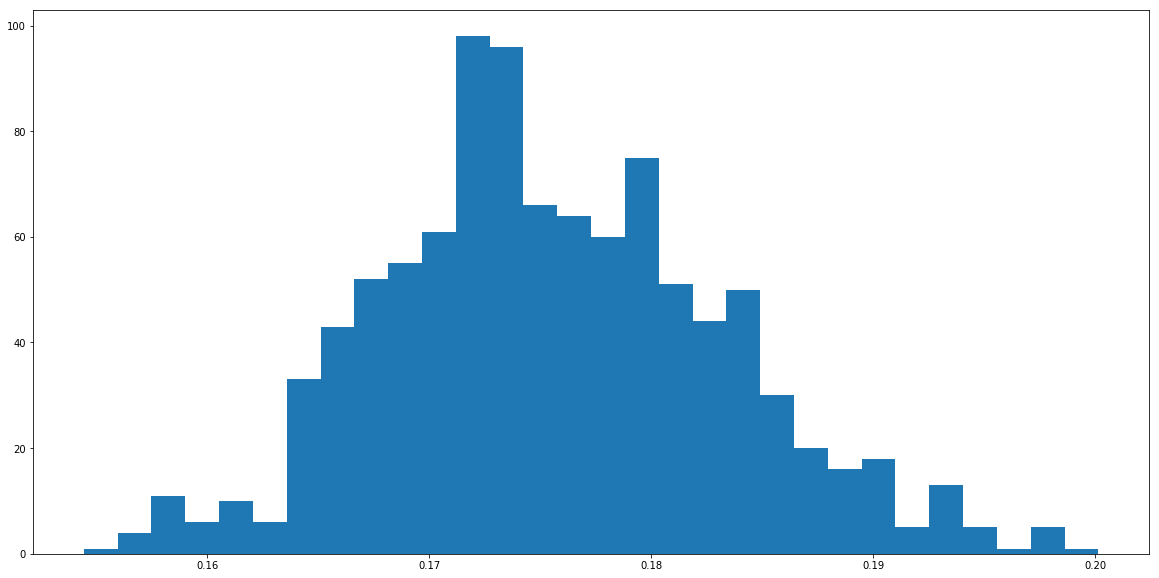Don’t let the rescaling plot fool you. This is a mighty thin posterior. We are more or less certain that the parameter is between 0.16 and 0.19.

And finally then, the HMC rankings, together with the real result and the SVI result.

In :
svid = {x: rank for rank, x in enumerate(svi_ranks)}
svi_results = [svid.get(team, -1) for team in ALLTEAMS]
mc_ranks = sorted(zip([round(sol.item()) for sol in marginal.mean],
ALLTEAMS,
TEAMRANKS,
svi_results), reverse=True)

pd.DataFrame(mc_ranks[:25],
columns=['Elo', 'Country', 'Final result', 'SVI result'])

Out:
Elo Country Final result SVI result
0 458 United States of America 1 0
1 449 Poland 3 2
2 448 Azerbaijan 14 1
3 436 China 0 3
4 415 France 8 4
5 412 India 5 6
6 412 Armenia 7 5
7 406 Russia 2 7
8 392 Ukraine 9 8
9 388 Vietnam 6 9
10 382 Czech Republic 11 10
11 367 England 4 11
12 358 Israel 38 12
13 358 Croatia 25 15
14 357 Netherlands 39 16
15 357 Germany 12 13
16 356 Uzbekistan 15 14
17 346 Spain 24 18
18 344 Iran 16 17
19 332 Georgia 1 27 19
20 325 Hungary 17 21
21 324 Romania 26 20
22 322 Sweden 10 22
23 313 Norway 28 23
24 305 Moldova 30 24

We can see that HMC and SVI give an almost identical mean. This is good. Two completely different methods tell us the same thing. The opinion club on Twitter was.. right?! Poland should have won a medal! Also, Azerbaijan were even more robbed, but for some reason there was no public outcry for them.

We can now look at and compare the world’s top posteriors.

In :
plt.figure(figsize=(20, 10))

teams = ['United States of America',
'Poland',
'Azerbaijan',
'China',
'Sweden']

dists = [[marginal.sample()[ALLTEAMS.index(team)] for _ in range(1000)]
for team in teams]
plt.hist(dists, bins=30, label=teams)
plt.legend()
plt.show()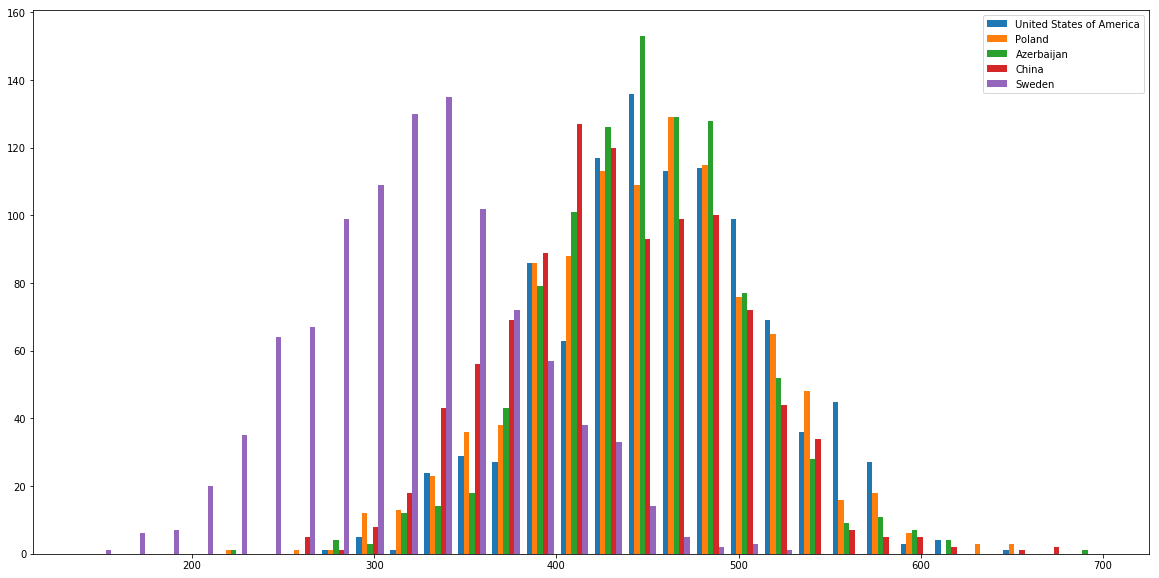Yeah, I could probably have visualized this better, but as you can see, they are almost identical. Sweden is clearly worse than the top teams, but even their distribution overlaps the top teams.

We can write a function that samples from the posteriors to see the probability that one team has a better true Elo than another.

In :
def is_better(c1, c2, iterations=10000):
i1 = ALLTEAMS.index(c1)
i2 = ALLTEAMS.index(c2)
samples = [marginal.sample() for _ in range(iterations)]
tsamples = torch.stack(samples, dim=1)
nr = (tsamples[i1] > tsamples[i2]).sum().item()
percent = round(nr*100/iterations)
print(f'{percent}% chance that {c1} was better than {c2}.')

is_better('United States of America', 'China')
is_better('United States of America', 'Poland')
is_better('United States of America', 'Sweden')

60% chance that United States of America was better than China.
55% chance that United States of America was better than Poland.
95% chance that United States of America was better than Sweden.


And, since the world needs to know.

In :
is_better('Sweden', 'Norway')

55% chance that Sweden was better than Norway.


Finally (for real this time), we can sample from the posterior and check the probability for each team, that they were in fact the best one.

In :
from collections import Counter

rks = [Counter() for _ in ALLTEAMS]
for i in range(10000):
rankings = zip([round(sol.item()) for sol in marginal.sample()],
ALLTEAMS,
range(len(ALLTEAMS)))
for j, (_, c, _) in enumerate(sorted(rankings, reverse=True)):
rks[j].update([c])

for country, nr in rks.most_common()[:10]:
print(f'{nr/100:.1f}% chance that {country} was the best team.')

21.1% chance that United States of America was the best team.
18.6% chance that Poland was the best team.
13.0% chance that Azerbaijan was the best team.
12.6% chance that China was the best team.
6.6% chance that France was the best team.
4.7% chance that Armenia was the best team.
4.0% chance that Russia was the best team.
3.6% chance that India was the best team.
3.3% chance that Vietnam was the best team.
2.6% chance that Ukraine was the best team.


## Conclusion

Pyro + Pytorch is really easy to use. You can define functions with no magic or decorators or anything like that. Just define the random process that you think generates the data that you see, and you can get the most likely parameters for this process.

Probabilistic programming is especially well suited for when you have many parameters and not so much data, giving you an opportunity to work with your prior knowledge and the uncertainty in the output.

### AuthorDavid Fendrich
CTO
David Fendrich is our Chief Technical Officer and one of the founders of Tenfifty. He has been working with AI and data science for almost two decades, helping companies put cutting edge solutions into practical use.
##### More from this author